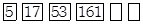# Test: Fun With Numbers - 1

## 10 Questions MCQ Test Mathematics (Maths) for Class 4 | Test: Fun With Numbers - 1

Description
This mock test of Test: Fun With Numbers - 1 for Class 4 helps you for every Class 4 entrance exam. This contains 10 Multiple Choice Questions for Class 4 Test: Fun With Numbers - 1 (mcq) to study with solutions a complete question bank. The solved questions answers in this Test: Fun With Numbers - 1 quiz give you a good mix of easy questions and tough questions. Class 4 students definitely take this Test: Fun With Numbers - 1 exercise for a better result in the exam. You can find other Test: Fun With Numbers - 1 extra questions, long questions & short questions for Class 4 on EduRev as well by searching above.
QUESTION: 1

### A and B are natural numbers. Value of A is twice of B. B is 244689 more than 654123. Find the successor of A.

Solution:

B = 244689 + 654123 = 898812

A = 2B = 1797624

successor of A = 1797624 + 1 = 1797625

QUESTION: 2

Solution:
QUESTION: 3

### Write the expanded form for numeral 7400546

Solution:
QUESTION: 4

Write the number, seventy five crore five lakh thirteen thousand two hundred nineteen, as per international system of numeration.

Solution:
QUESTION: 5

How many times does the place value of 8 in the numeral 2583219705 is greater than the face value of 8?

Solution:

the place value of 8 in 2583219705 is

8 x 10000000 = 80000000

face value of 8 = 8

so 80000000 is 10000000 times more than 8

QUESTION: 6

In the numeral 45695A221442, name the period that A occupies.

Solution:
QUESTION: 7

We can arrange the numbers in many specific way. Ascending and descending order of numbers are also a kind of arrangement of numbers, In descending order numbers are arranged from.

Solution:
QUESTION: 8

Which one of the following is not true.

Solution:
QUESTION: 9

Jack selects the number 542631 and finds its predecessor. If he increases the number by 2348795, by how much the predecessor will increase.

Solution:
QUESTION: 10

Complete the following number series.Solution:

5 = 1 + 4

17 = 5 + 12

53 = 17 + 36

161 = 53 + 108

485 = 161 + 324

1457 = 485 + 972